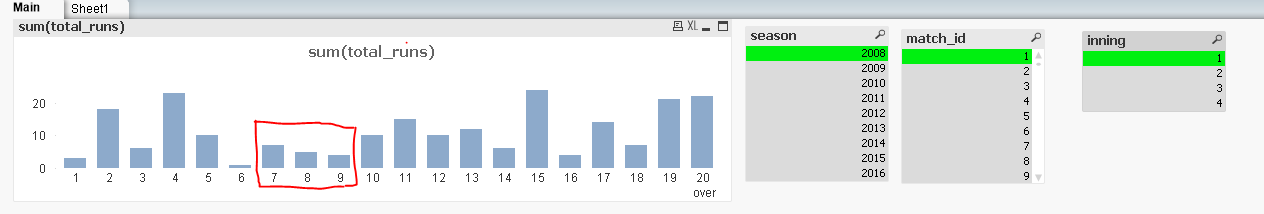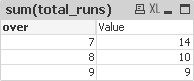# QlikView App Dev

Discussion Board for collaboration related to QlikView App Development.

Announcements
On May 18th at 10AM EDT we will answer your QlikView questions live. REGISTER
cancel
Showing results for
Did you mean:Specialist

## Need to find number of wickets fallen/dismissed in between the period of any three consecutive overs (per innings) where runs decreased gradually ?

Hi All ,

How to find number of wickets fallen in between the period of any three consecutive overs where runs decreased gradually ?In season 2008 match id 1 , in the 1st inning , over 7 , 8 and 9 witnessed gradual decrease in RUNS . I need to find number of wicket fallen in between this period .

Note : Field  " dismissal_kind " ... gives fall of wicket .

Thanks & Regards

Shekar

1 Solution

Accepted SolutionsMaster

Try:

Over Count(player_dismissed)
6727
Not Declining5005
Runs Declining1722

Where Over is the calculated dimension:

=Aggr(

if(

Sum(total_runs) < Above(Sum (total_runs)) AND Above(Sum (total_runs))<Above(Sum (total_runs),2)

OR

Sum(total_runs) < Above(Sum (total_runs)) AND Below(Sum (total_runs))<Sum (total_runs)

OR

Below(Sum (total_runs)) < Sum(total_runs)AND Below(Sum (total_runs),2)<Below(Sum (total_runs))

, 'Runs Declining', 'Not Declining'),over)

Regards

Andrew

10 RepliesSpecialist
Author

stalwar1‌   .... your expertise needed .Specialist
AuthorSpecialist
Author

stalwar1‌ ... Hi Sunny .. please let me know if this requirement is unrealistic and not possibleMaster

Hi Shektar,

Try this for the bar background colour:

if(

Sum(total_runs) < Above(Sum (total_runs)) AND Above(Sum (total_runs))<Above(Sum (total_runs),2)

OR

Sum(total_runs) < Above(Sum (total_runs)) AND Below(Sum (total_runs))<Sum (total_runs)

OR

Below(Sum (total_runs)) < Sum(total_runs)AND Below(Sum (total_runs),2)<Below(Sum (total_runs))

, LightRed())

givingRegards

AndrewMVP

Next time, maybe you should include the rules of the game for cricket. Not really a major sport in either the US or Italy, I guessSpecialist
Author

Hi Andrew ,

Thanks for the reply , but its not the background colour i was looking for but wanted to find no. of players dismissed between those overs which has gradual decrease in runs for three consecutive overs .

Note : Actually there has been an analysis in cricket that , chances of dismissal increases when a bowler bowls continuous 3-4 overs with less runs . I just wanted to test that .

Thanks & Regards

shekharMaster

Try:

Over Count(player_dismissed)
6727
Not Declining5005
Runs Declining1722

Where Over is the calculated dimension:

=Aggr(

if(

Sum(total_runs) < Above(Sum (total_runs)) AND Above(Sum (total_runs))<Above(Sum (total_runs),2)

OR

Sum(total_runs) < Above(Sum (total_runs)) AND Below(Sum (total_runs))<Sum (total_runs)

OR

Below(Sum (total_runs)) < Sum(total_runs)AND Below(Sum (total_runs),2)<Below(Sum (total_runs))

, 'Runs Declining', 'Not Declining'),over)

Regards

AndrewSpecialist II

Maybe something like the below

if( (over >=sum(total aggr(DISTINCT  if ( (rangesum(Above( (sum(total_runs) <  Above(sum(total_runs))) ,0,2))*-1 )=2 and
(rangesum(Above( (sum(total_runs) - Above(sum(total_runs)) ) ,0,2))*-1 )<15 , over ),over ))-2 )   and

( over<=sum(total aggr(DISTINCT  if ( (rangesum(Above( (sum(total_runs) <  Above(sum(total_runs))) ,0,2))*-1 )=2 and
(rangesum(Above( (sum(total_runs) - Above(sum(total_runs)) ) ,0,2))*-1 )<15 , over ),over )) )

, sum(total_runs))Specialist
Author

Hi Andrew ,

Your solution worked perfectly and the colour coding which you had given earlier is like bonus . Just little add on it ..

How to set reference line in the highlighted over where a player got dismissed .
Example in 6th over player SC ganguly got dismissed